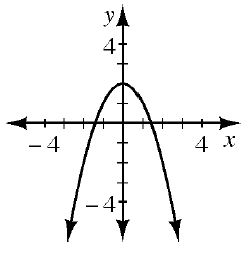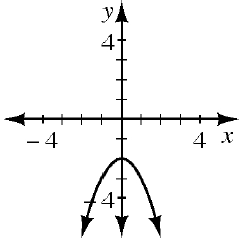### Home > CAAC > Chapter 8 > Lesson 8.1.4 > Problem8-42

8-42.

Match each rule below with its corresponding graph. Can you do this without making any tables? Explain your selections.

1. $y=−x^2−2$

1. $y=x^2−2$

1. $y=−x^2+2$

1.1.1.Determine the direction of the opening and $y$-intercept for each of the given equations.

$2$